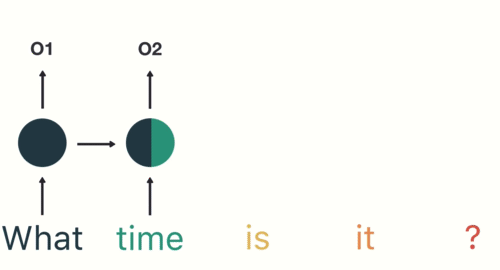# Depth-Gated LSTM: From A to Z!Depth-Gated LSTM: From A to Z! Recurrent Neural Networks(RNN) suffer from short-term memory. This means that if there is a long sequence, an RNN will have a problem in carrying information from earlier time steps to later ones.

Recurrent Neural Networks(RNN) suffer from short-term memory. This means that if there is a long sequence, an RNN will have a problem in carrying information from earlier time steps to later ones. Which may force the RNN to leave out important information from the beginning.

In-depth, during backpropagation, recurrent neural networks suffer from the vanishing of its gradients(Gradients are values used to update neural network weights)this problem occurs when the gradient shrinks as it back propagates through time.

## But First, What is an RNN?

Recurrent Neural Network(RNN) is a type of Neural Network where the output from the previous step is fed as input to the current step. In other words, it converts the independent activations into dependent ones by providing the same weights and biases to all the layers, thus reducing the complexity of increasing parameters and memorizing each previous outputs by giving each output as input to the next hidden layer.

## The Recurrent Neural Network (RNNs)

A recurrent neural network is an input node that feeds sigmoid activation. The way an RNN does this is to take the output of one neuron and return it as input to another neuron or feed the input of the current time step to the output of earlier time steps. Here you feed the input from the previous times step by step into the input of the current times and vice versa.

## Recurrent Neural Network with LSTM

Recurrent Neural Network with LSTM. Starting from scratch, this blog will help you knowing RNN at its peak in the easiest way along with the importance of LSTM.

## All About Artificial Neural Networks – What, Why, and How?

Artificial Neural Network is a learning system that seeks to emulate the human brain. Here is an overview of ANN, critical to the discipline of Artificial Intelligence.

## Introduction to Artificial Neural Networks for Beginners

Introduction to Artificial Neural Networks for Beginners. Understanding the concepts of Neural Networks.

## Artificial Neural Networks — Recurrent Neural Networks

Artificial Neural Networks — Recurrent Neural Networks. Remembering the history and predicting the future with neural networks. A intuition behind Recurrent neural networks.